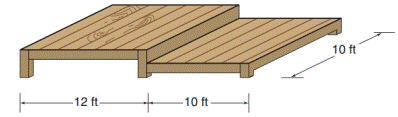Chapter 8.1, Problem 28E### Elementary Geometry for College St...

6th Edition
Daniel C. Alexander + 1 other
ISBN: 9781285195698

#### Solutions

Chapter
Section### Elementary Geometry for College St...

6th Edition
Daniel C. Alexander + 1 other
ISBN: 9781285195698
Textbook Problem
1 views

# Gary and Carolyn plan to build the deck shown.a) Find the total floor space (area) of the deck.b) Find the approximate cost of building the deck if the estimated cost is $6.40 per ft 2 .To determine a) To find: The total floor space (area) of the deck. Explanation Converting theoretical statement into mathematical statement, identifying the figure and the calculation is followed by suitable geometric formulae. Calculation: Given, Length of rectangular deck floor space l=(12+10)=22 ft Width of the rectangular deck floor space w=10 ft Total area of the rectangular deck To determine b) To find: The approximate cost of building the deck if the estimated cost is$6.40 per ft2.

### Still sussing out bartleby?

Check out a sample textbook solution.

See a sample solution

#### The Solution to Your Study Problems

Bartleby provides explanations to thousands of textbook problems written by our experts, many with advanced degrees!

Get Started

#### In Exercises 1 and 2, simplify the expression. 5(2)34

Calculus: An Applied Approach (MindTap Course List)

#### Simplify: 1216

Elementary Technical Mathematics

#### Evaluate the expression sin Exercises 116. (23)2

Finite Mathematics and Applied Calculus (MindTap Course List)

#### For

Study Guide for Stewart's Multivariable Calculus, 8th